Question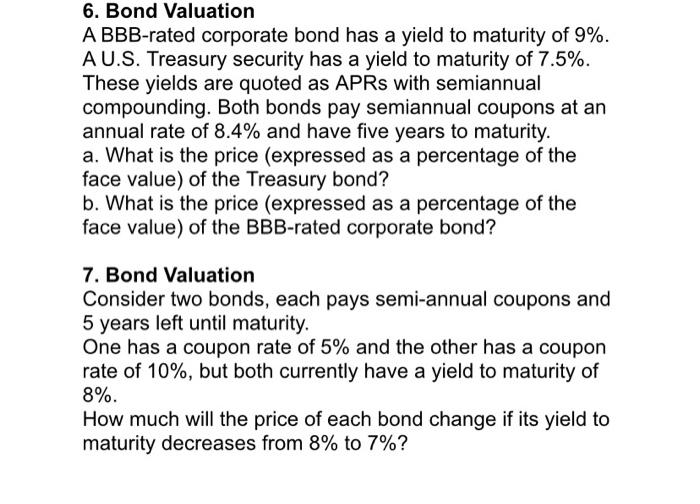Question 6:

For both the bonds assuming Face value = 1K, PMT i.e. coupon = 48 @ 8.4% semiannually(8.4% / 2) , Number of payments = 10 (5 yrs semiannual, 5*2)

For BBB rated corporate bond I/Y = 9 / 2 (semiannual) therefore price 976.261

For Treasury bond I/Y = 7.5 / 2 (semiannual) therefore price 1036.95

Question 7:

For both the bonds assuming Face value = 1K, Number of payments = 10 (5 yrs semiannual, 5*2)

Bond with coupon rate 5%

PMT = 25 (5% of 1000 = 50 / 2 aince coupon is semiannual)

When,

I/Y = 8 / 2 (Semiannual) , price = 878.33

I/Y = 7 / 2 (Semiannual), price = 916.83

Price difference = 916.83-878.33 = 38.50

Bond with coupon rate 10%

PMT = 50(10% of 1000 = 100 / 2 since coupon is semiannual)

When,

I/Y = 8 / 2 (Semiannual) , price = 1081.10

I/Y = 7 / 2 (Semiannual), price = 1124.74

Price difference = 1124.74 - 1081.10 = 43.63

#### Earn Coins

Coins can be redeemed for fabulous gifts.

Similar Homework Help Questions
• ### A BBB-rated corporate bond has a yield to maturity of 7.9%. AU.S. Treasury security has a...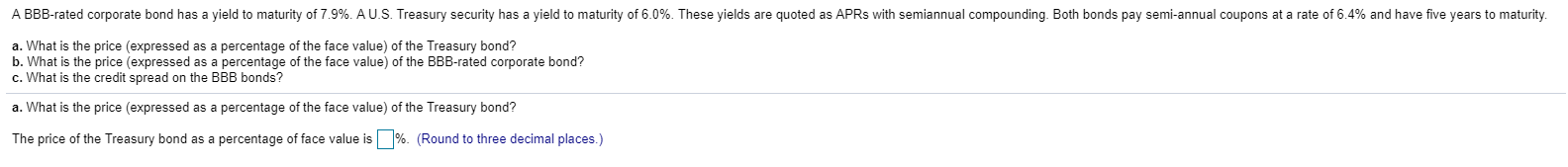A BBB-rated corporate bond has a yield to maturity of 7.9%. AU.S. Treasury security has a yield to maturity of 6.0%. These yields are quoted as APRs with semiannual compounding. Both bonds pay semi-annual coupons at a rate of 6.4% and have five years to maturity. a. What is the price (expressed as a percentage of the face value) of the Treasury bond? b. What is the price (expressed as a percentage of the face value) of the BBB-rated corporate...

• ### A​ BBB-rated corporate bond has a yield to maturity of 6.3 % A U.S. treasury security...

A​ BBB-rated corporate bond has a yield to maturity of 6.3 % A U.S. treasury security has a yield to maturity of 4.3 % These yields are quoted as APRs with semiannual compounding. Both bonds pay​ semi-annual coupons at a rate of 5.0 % and have five years to maturity.     a. What is the price​ (expressed as a percentage of the face​ value) of the treasury​ bond? b. What is the price​ (expressed as a percentage of the face​ value)...

• ### A​ BBB-rated corporate bond has a yield to maturity of 10.8 %. A U.S. treasury security...

A​ BBB-rated corporate bond has a yield to maturity of 10.8 %. A U.S. treasury security has a yield to maturity of 9.5 %. These yields are quoted as APRs with semiannual compounding. Both bonds pay​ semi-annual coupons at a rate of 10.1 % and have five years to maturity.     a. What is the price​ (expressed as a percentage of the face​ value) of the treasury​ bond? b. What is the price​ (expressed as a percentage of the face​ value)...

• ### A​ BBB-rated corporate bond has a yield to maturity of 6.4%. A U.S. treasury security has a yield to maturity of 4.4%. T...

A​ BBB-rated corporate bond has a yield to maturity of 6.4%. A U.S. treasury security has a yield to maturity of 4.4%. These yields are quoted as APRs with semiannual compounding. Both bonds pay​ semi-annual coupons at a rate of 4.7% and have five years to maturity. a. What is the price​ (expressed as a percentage of the face​ value) of the treasury​ bond? b. What is the price​ (expressed as a percentage of the face​ value) of the​ BBB-rated...

• ### A BBB-rated corporate bond has a yield to maturity of 11.8%. A U.S. Treasury security has a yield to maturity of 10.5%. These yields are quoted as APRs with semiannual compounding. Both bonds pay semiannual coupons at an annual rate of 11.0% and have five

A BBB-rated corporate bond has a yield to maturity of 11.8%. A U.S. Treasury security has a yield to maturity of 10.5%. These yields are quoted as APRs with semiannual compounding. Both bonds pay semiannual coupons at an annual rate of 11.0% and have five years to maturity.      a. What is the price (expressed as a percentage of the face value) of the Treasury bond? b. What is the price (expressed as a percentage of the face value) of the BBB-rated corporate bond? c. What is the credit...

• ### A BBB-rated corporate bond has a yield to maturity of 11.8%. An Australian treasury security has...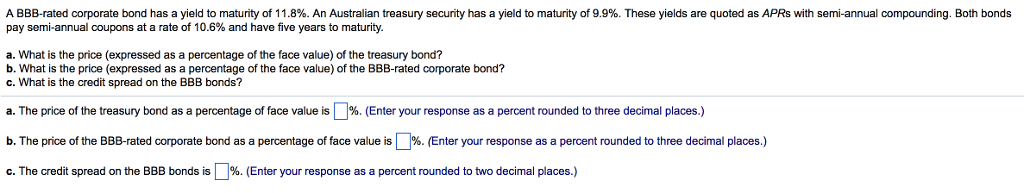A BBB-rated corporate bond has a yield to maturity of 11.8%. An Australian treasury security has a yield to maturity of 9.9%. These yields are quoted as APRs with semi-annual compounding. Both bonds pay semi-annual coupons at a rate of 10.6% and have five years to maturity. a. What is the price (expressed as a percentage of the face value) of the treasury bond? b. What is the price (expressed as a percentage of the face value) of the BBB-rated...

• ### Thank you! Problem 6-30 A BBB-rated corporate bond has a yield to maturity of 8.2%. A...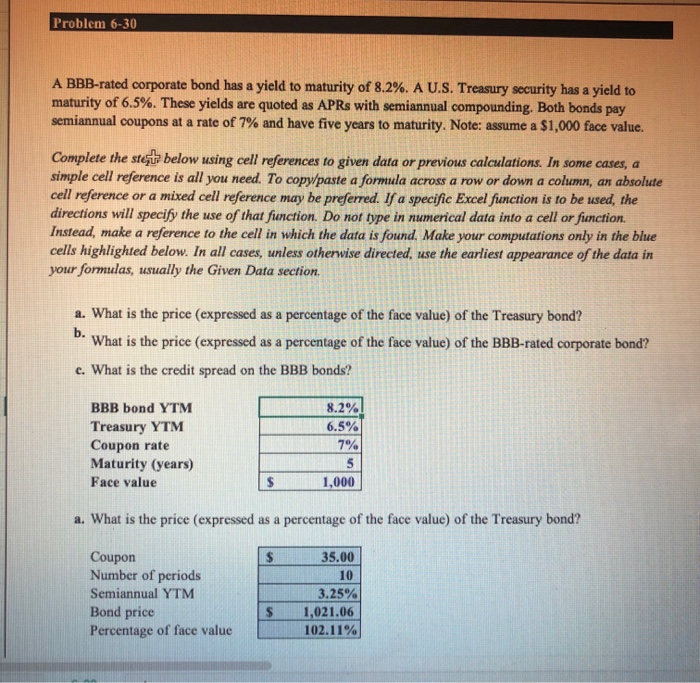Thank you! Problem 6-30 A BBB-rated corporate bond has a yield to maturity of 8.2%. A U.S. Treasury security has a yield to maturity of 6.5%. These yields are quoted as APRs with semiannual compounding. Both bonds pay semiannual coupons at a rate of 7% and have five years to maturity. Note: assume a \$1,000 face value. Complete the stata below using cell references to given data or previous calculations. In some cases, a simple cell reference is all you...

• ### 3. Interest Rates You have just purchased a home and taken out a \$460,000 mortgage. The...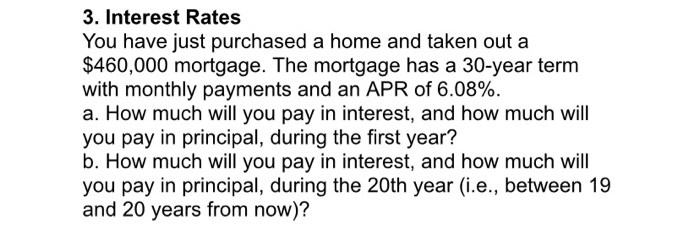3. Interest Rates You have just purchased a home and taken out a \$460,000 mortgage. The mortgage has a 30-year term with monthly payments and an APR of 6.08%. a. How much will you pay in interest, and how much will you pay in principal, during the first year? b. How much will you pay in interest, and how much will you pay in principal, during the 20th year (i.e., between 19 and 20 years from now)? 6. Bond Valuation...

• ### 2. Interest Rates You are saving for retirement. To live comfortably, you decide you will need...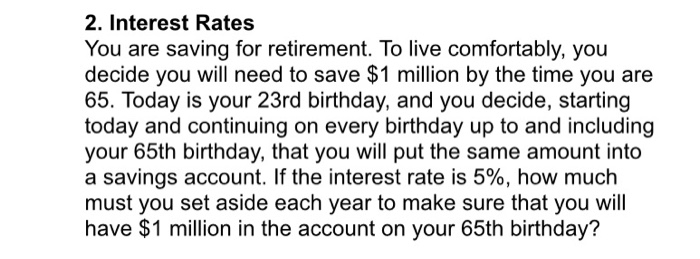2. Interest Rates You are saving for retirement. To live comfortably, you decide you will need to save \$1 million by the time you are 65. Today is your 23rd birthday, and you decide, starting today and continuing on every birthday up to and including your 65th birthday, that you will put the same amount into a savings account. If the interest rate is 5%, how much must you set aside each year to make sure that you will have...

• ### Suppose that General Motors Acceptance Corporation issued a bond with 10 years until maturity, a face...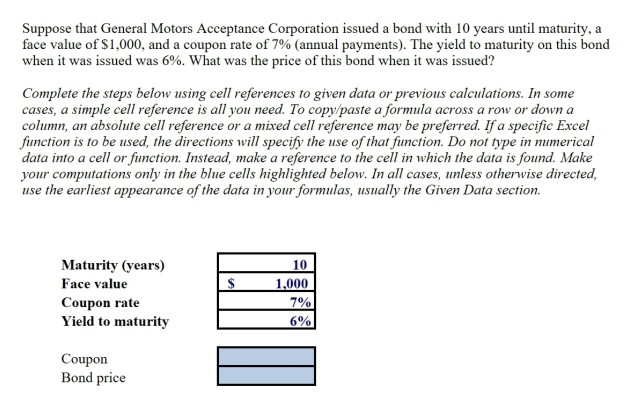Suppose that General Motors Acceptance Corporation issued a bond with 10 years until maturity, a face value of \$1,000, and a coupon rate of 7% (annual payments). The yield to maturity on this bond when it was issued was 6%. What was the price of this bond when it was issued? Complete the steps below using cell references to given data or previous calculations. In some cases, a simple cell reference is all you need. To copy paste a formula...